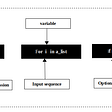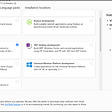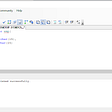# Export model list to Excel in C# (Using Interop) — The easy way!

`public class UserManager{    public string Id { get; set; }    public string Username { get; set; }    public string FirstName { get; set; }    public string LastName { get; set; }    public string Password { get; set; }    public DateTime CreatedOn { get; set; }}`
`public static List<UserManager> SeedData(){ return new List<UserManager> {  new UserManager  {   Id = Guid.NewGuid().ToString(),   FirstName = "Test 1",   LastName = "Test 1",   Username = "TestUsername1",   Password = "123",   CreatedOn = DateTime.UtcNow  },  new UserManager  {   Id = Guid.NewGuid().ToString(),   FirstName = "Test 2",   LastName = "Test 2",   Username = "TestUsername2",   Password = "123",   CreatedOn = DateTime.UtcNow  },  new UserManager  {   Id = Guid.NewGuid().ToString(),   FirstName = "Test 3",   LastName = "Test 3",   Username = "TestUsername3",   Password = "123",   CreatedOn = DateTime.UtcNow  },new UserManager  {   Id = Guid.NewGuid().ToString(),   FirstName = "Test 4",   LastName = "Test 4",   Username = "TestUsername4",   Password = "123",   CreatedOn = DateTime.UtcNow  } };}`
`// T is a generic classstatic DataTable ConvertToDataTable<T>(List<T> models){ // creating a data table instance and typed it as our incoming model  // as I make it generic, if you want, you can make it the model typed you want. DataTable dataTable = new DataTable(typeof(T).Name);//Get all the properties of that model PropertyInfo[] Props = typeof(T).GetProperties(BindingFlags.Public | BindingFlags.Instance); // Loop through all the properties             // Adding Column name to our datatable foreach (PropertyInfo prop in Props) {  //Setting column names as Property names    dataTable.Columns.Add(prop.Name); } // Adding Row and its value to our dataTable foreach (T item in models) {  var values = new object[Props.Length];  for (int i = 0; i < Props.Length; i++)  {   //inserting property values to datatable rows     values[i] = Props[i].GetValue(item, null);  }  // Finally add value to datatable    dataTable.Rows.Add(values); } return dataTable;}`
`public static void GenerateExcel(DataTable dataTable, string path){}`
`public static void GenerateExcel(DataTable dataTable, string path){  DataSet dataSet = new DataSet(); dataSet.Tables.Add(dataTable);}`
`using Excel = Microsoft.Office.Interop.Excel;public static void GenerateExcel(DataTable dataTable, string path){  DataSet dataSet = new DataSet(); dataSet.Tables.Add(dataTable);// create a excel app along side with workbook and worksheet and give a name to it Excel.Application excelApp = new Excel.Application(); Excel.Workbook excelWorkBook = excelApp.Workbooks.Add(); Excel._Worksheet xlWorksheet = excelWorkBook.Sheets; Excel.Range xlRange = xlWorksheet.UsedRange; foreach (DataTable table in dataSet.Tables) {  //Add a new worksheet to workbook with the Datatable name  Excel.Worksheet excelWorkSheet = excelWorkBook.Sheets.Add();  excelWorkSheet.Name = table.TableName;   // add all the columns  for (int i = 1; i < table.Columns.Count + 1; i++)  {   excelWorkSheet.Cells[1, i] = table.Columns[i - 1].ColumnName;  }  // add all the rows  for (int j = 0; j < table.Rows.Count; j++)  {   for (int k = 0; k < table.Columns.Count; k++)   {    excelWorkSheet.Cells[j + 2, k + 1] =       table.Rows[j].ItemArray[k].ToString();   }  } }}`
`// excelWorkBook.Save(); -> this will save to its default locationexcelWorkBook.SaveAs(path); // -> this will do the customexcelWorkBook.Close();excelApp.Quit();`
`static void Main(string[] args){ var userList = UserManager.SeedData(); try {  Console.WriteLine("Please select a operation to do ..");  Console.WriteLine("1. Export Data as EXCEL");  int command = Convert.ToInt32(Console.ReadLine());  switch (command)  {   case 1:    string fileName = "UserManager.xlsx";    Console.WriteLine("Please give a location to save :");    string location = Console.ReadLine();    string customExcelSavingPath = location + "\\" + fileName;    ExcelExport.GenerateExcel(ConvertToDataTable(userList), customExcelSavingPath);    break;   default:    break;  } } catch (Exception ex) {  throw ex; }}`

--

--

--

## More from Pritom Purkayasta

if you like the content, you know what to do 🎉✔

Love podcasts or audiobooks? Learn on the go with our new app.

## Google playstore Errors & Solutions on Huawei P10 Plus## A walk through python List Comprehension## Day 2 - 29th May, 2020 - Hybrid Multi Cloud By Mr Vimal Daga from Linux World India Pvt Ltd## I want to contribute to open source## Top 5 services for developers to show their web projects for FREE## Starter Bootstrap Theme## Pritom Purkayasta

if you like the content, you know what to do 🎉✔

## Unit Tests with C#, NUnit Framework and FluentAssertions.## Backend and Frontend Walking Skeleton## MAUI: Hello World## Types Of View in SQL Server